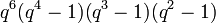# Linear representation theory of special linear group over a finite field

## Contents

This article gives specific information, namely, linear representation theory, about a family of groups, namely: special linear group. This article restricts attention to the case where the underlying ring is a finite field.
View linear representation theory of group families | View other specific information about special linear group | View other specific information about group families for rings of the type finite field

## Particular cases

### Particular cases by degre

Value of degree$n$ Linear representation theory of
special linear group$SL(n,q)$
order of group Degree as a polynomial in$q$ (=$n^2 - 1$) Number of irreducible representations equals number of conjugacy classes (see number of conjugacy classes in special linear group of fixed degree over a finite field is PORC function of field size)
1 it is the trivial group 1 0 1
2 link$q^3 - q$ 3$q + 1$ if$q$ even$q + 4$ if$q$ odd
3 link$q^3(q^3 - 1)(q^2 - 1)$ 8$q(q + 1)$ if$q$ not 1 mod 3$q^2 + q + 8$ if$q$ is 1 mod 3
4 link$q^6(q^4 - 1)(q^3 - 1)(q^2 - 1)$ 15$q^3 + q^2 + q$ if$q$ even$q^3 + q^2 + 4q + 3$ if$q$ is 3 mod 4$q^3 + q^2 + 4q + 15$ if$q$ is 1 mod 4